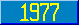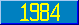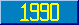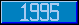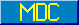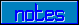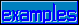## Number of Subscripts

### 2.4.2 Number of subscripts

The number of subscripts in a global variable is limited in that, in a global array reference, the total length of the array reference must not exceed the maximum string length (see 2.8). The length of an array reference is calculated as follows:

assuming an array reference in the form:

^|environment|name(i1,i2, ... ,in)
E = \$L(environment)
N = \$L(name)
I = \$L(i1) + \$L(i2) + ... + \$L(in)

where each subscript (i1 through in) is either a numlit or a sublit

L = n

then the total length of an array reference is

E + 3 + N + I + ( 2 * L ) + 15